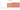# د.ل.‏

## Libyan Dinar

Libya's national currency is the dinar. One thousand dirhams equal one dinar. It is issued by the Libyan Central Bank, which also controls credit and oversees the banking industry.

#### how has the value of the currency changed in the last year?## USD 1 = LYD 4.8406

How much is10 US dollars worth inLibyan dinars?
At the current exchange rate, 10 US dollars is worth 48.41 Libyan dinars
How much is50 US dollars worth inLibyan dinars?
At the current exchange rate, 50 US dollars is worth 242.03 Libyan dinars
How much is100 US dollars worth inLibyan dinars?
At the current exchange rate, 100 US dollars is worth 484.06 Libyan dinars
How much is500 US dollars worth inLibyan dinars?
At the current exchange rate, 500 US dollars is worth 2,420.3 Libyan dinars
How much is2,000 US dollars worth inLibyan dinars?
At the current exchange rate, 2,000 US dollars is worth 9,681.21 Libyan dinars
How much is10 US dollars worth inLibyan dinars?
At the current exchange rate, 10 US dollars is worth 48.41 Libyan dinars
How much is50 US dollars worth inLibyan dinars?
At the current exchange rate, 50 US dollars is worth 242.03 Libyan dinars
How much is100 US dollars worth inLibyan dinars?
At the current exchange rate, 100 US dollars is worth 484.06 Libyan dinars
How much is500 US dollars worth inLibyan dinars?
At the current exchange rate, 500 US dollars is worth 2,420.3 Libyan dinars
How much is2,000 US dollars worth inLibyan dinars?
At the current exchange rate, 2,000 US dollars is worth 9,681.21 Libyan dinars